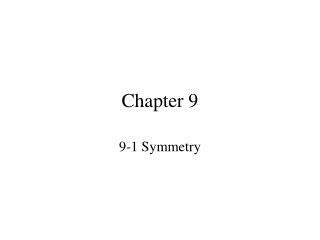DownloadDownload PresentationChapter 9

# Chapter 9

Télécharger la présentation## Chapter 9

- - - - - - - - - - - - - - - - - - - - - - - - - - - E N D - - - - - - - - - - - - - - - - - - - - - - - - - - -
##### Presentation Transcript

1. Chapter 9 9-1 Symmetry

2. Determine if the function is odd even or neither

3. HW #9.1Pg 389-390 1-33 Odd 35-62

4. Chapter 9 9-2 Translations

5. An alteration of a relation is called a transformation. Moving the graph of a relation without changing the shape or size is called a translation

6. Consider the graph of y = |x|, sketch the graphs of the following by translating

7. Given the graph of y = x2 use transformations to sketch the graph of y = (x + 3)2 - 5

8. The graph of a function is given, sketch the graph under the given translations (c) Q(x) = f(x – 3) + 2

9. HW #9.2Pg 393 1-28, 29-39 Odd

10. Chapter 9 9-3 Stretching and Shrinking

11. Multiplying the function causes the graph to stretch or shrink vertically

12. The negative causes a reflection over the x-axis

13. The negative causes a reflection over the x-axis

14. The negative causes a reflection over the x-axis

15. The graph of f(x) is given use the graph to find the graph of y = 2f(x) The graph of y = 2f(x) is obtained by multiplying each y-value by 2

16. To get the same y-values, the x-values must be smaller as in Y2 larger as in Y3

17. Opposite x-values have the same y-values

18. Opposite x-values have the same y-values

19. Opposite x-values have the same y-values

20. Find the function that is finally graphed after the series of transformations are applied to the graph of

21. c d c a

22. HW# 9.3Pg 398-399 1-25 Odd, 26-53

23. Chapter 9 9-5 Graphs of Quadratic Functions

24. A quadratic function has the form y = ax2 + bx + cwhere a 0.

25. The graph is “U-shaped” and is called a parabola.

26. The highest or lowest point on the parabola is called the vertex.

27. In general, the axis of symmetry for the parabola is the vertical line through the vertex.

28. Sketch the graph of the following find the vertex, line of symmetry and the maximum or minimum value: 30. y < x2 31. y  x2 32. y  -3(x + 3)2

29. Graphing a Quadratic Function Graphing a Quadratic Function 1 Graph y= – (x+ 3)2 + 4 2 (–3, 4) 1 a = – , h = –3, and k = 4 2 SOLUTION The function is in vertex form y = a(x – h)2 +k. a < 0, the parabola opens down. To graph the function, first plot the vertex(h, k) = (–3, 4).

30. Graphing a Quadratic Function Graphing a Quadratic Function in Vertex Form 1 Graph y= – (x+ 3)2 + 4 2 (–1, 2) (–3, 4) Draw the axis of symmetryx = –3. (–5, 2) Plot two points on one side of it, such as (–1, 2) and (1, –4). Use symmetry to completethe graph. (1, –4) (–7, –4)

31. Sketch the graph of the following find the vertex, line of symmetry and the maximum or minimum value: